• Call Now

1800-102-2727•

# Nuclear fission-Explanation, Diagram, Examples, Practice problems, FAQs

Let me ask you a question: What would you do if you were so angry? You would explode into tears or yell at someone. Human beings, when they are overwhelmed, dissociate. A similar comparison can be made between unstable radioactive nuclei dissociating and human beings dissociating when they are overcome with emotions. For instance, Uranium U-235 is a radioactive nucleus–which upon being bombarded, dissociates into Krypton and Barium. The term “fission” refers to the breaking of a nucleus. Although it releases less energy compared to fusion, it is still very devastating. Such reactions are used in producing large quantities of heat in nuclear reactors. The stability of a radioactive nucleus is measured by its binding energy (BE) per nucleon–the higher the BE per nucleon, the higher the stability. In other words, to achieve higher BE per nucleon, the nuclei undergo fission. In this article, we will explore nuclear fission in detail.

• Definition of nuclear fission
• Nuclear chain reaction
• Energy released from each fission
• Classification of nuclear chain reaction
• Nuclear reactor
• Practice problems
• FAQs

## Definition of nuclear fission

When a heavy nucleus splits into two lighter nuclei, such a reaction is called nuclear fission. A good example would be the fission reaction of U235 which when bombarded with a neutron forms Barium and Krypton. The daughter nuclei thus formed have higher binding energy per nucleon compared to the parent nucleus.

${}_{92}{U}^{235}{+}_{0}{n}^{1}$=${}_{92}{U}^{236}$= ${}_{56}{Ba}^{141}+$${}_{36}{Kr}^{92}+{3}_{0}{n}^{1}+$

In the above reaction, an average of 2.5 neutrons are released. The 200 MeV (Mega Electron Volt) energy comes from the fact that mass defect is capable of being converted into energy. (Einstein’s mass-energy equivalence). The decay process is almost quite spontaneous and can be simulated in a lab.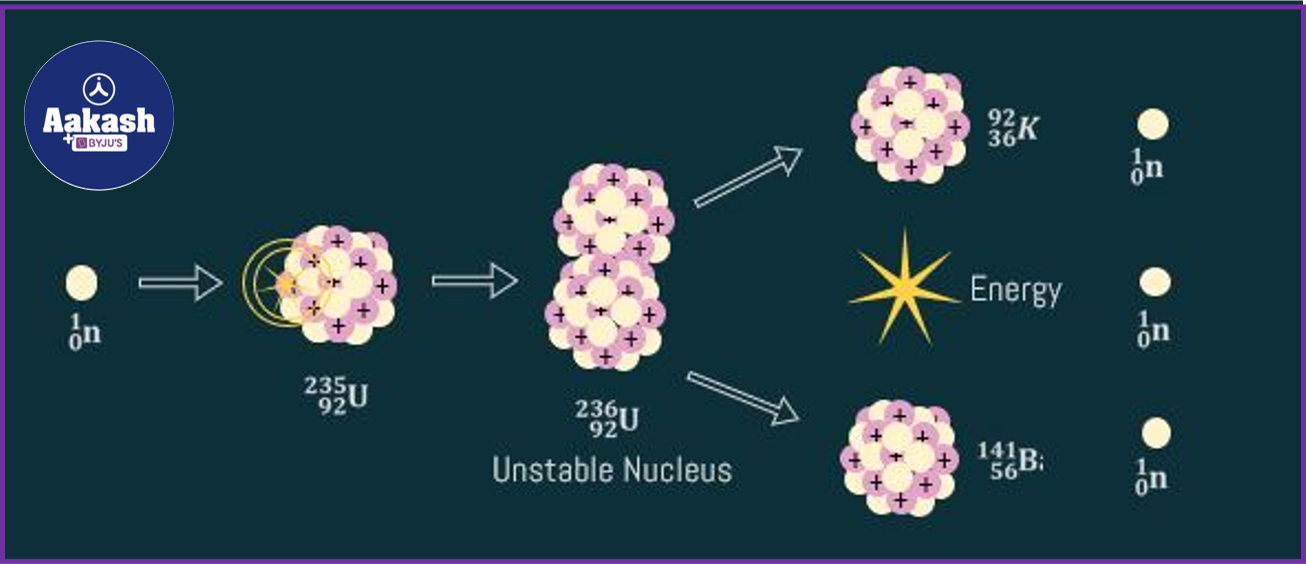Apart from Barium and Krypton, the following products are also possible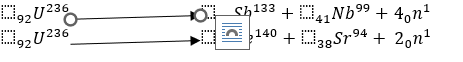Nuclear chain reaction

An accelerated neutron 0n1 upon colliding with the Uranium nucleus produces 3 more neutrons in the first generation. These 3 neutrons will bring about fissions which produce 3 more neutrons each, hence producing a total of 9 neutrons in the second generation. Hence, the number of neutrons produced in the nth generation=3n. Nuclear chain reactions needs to be controlled properly and also the fuel runs out quickly.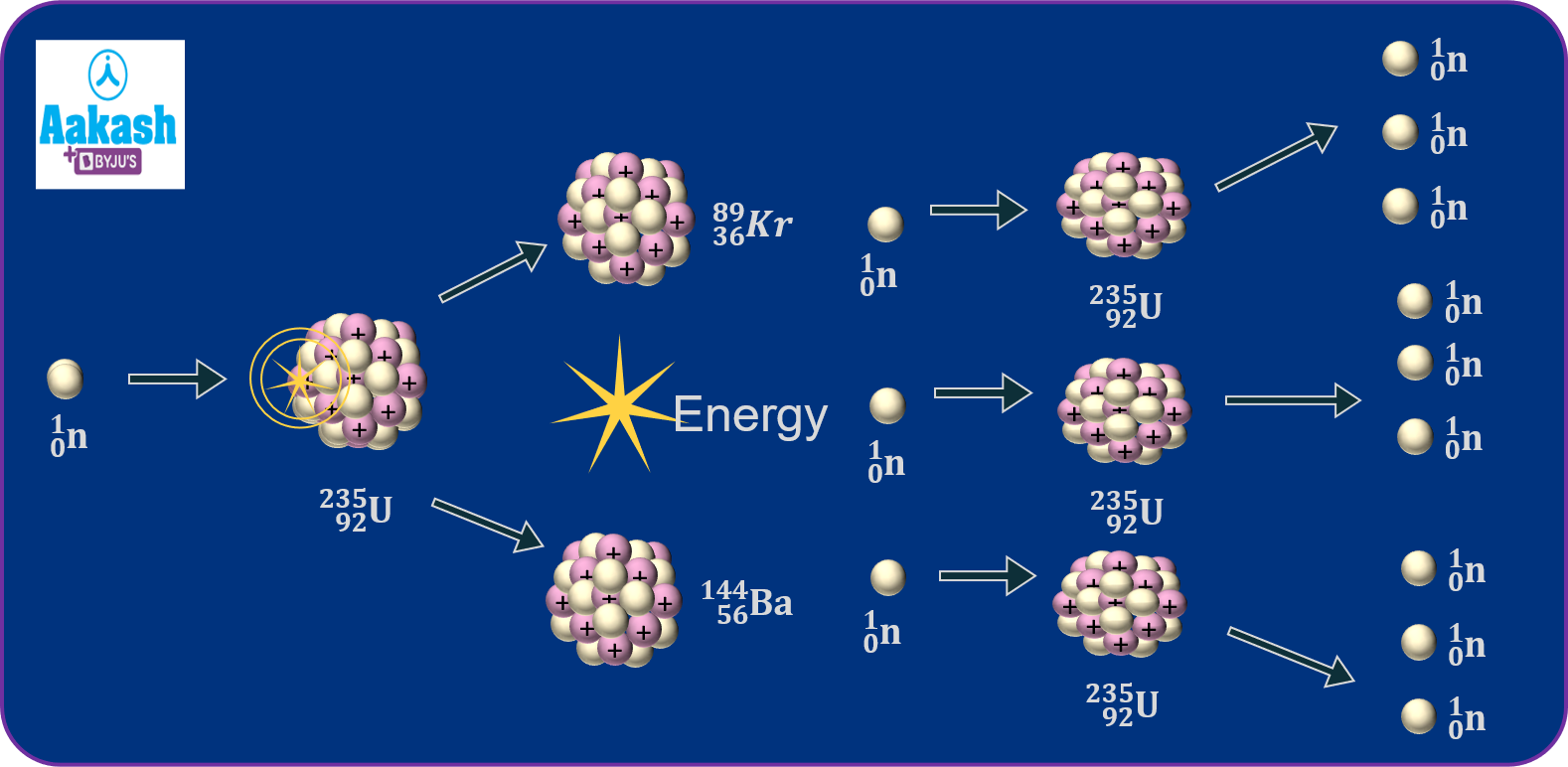Energy released from each fission

1) Kinetic energy of fission products-165 MeV

2) Gamma rays-7 MeV

3) Kinetic energy of the neutrons-6 MeV

4) Energy from fission products-7 MeV

5) Gamma rays from fission products-6 MeV

6) Anti-neutrons from fission products-9 MeV

Total energy =200 MeV.

## Classification of nuclear chain reaction

Uncontrolled nuclear reaction

Controlled nuclear reaction

When fission sets off more than one fission reaction, it is said to be uncontrolled.

When fission sets off only one fission reaction, it is said to be controlled.

Energy will multiply in milliseconds and finally result in an explosion.

Energy is liberated at a constant rate.

Example: Nuclear bomb

Example: Nuclear reactor

Nuclear reactor

Nuclear reactors, such as the ones located at Kalpakkam and Kudankulam, make use of nuclear fission to produce heat. The heat is in turn used to evaporate water which is used to run turbines. The multiplication factor K is defined as,

K=

When K>1, the reaction becomes very critical.

When K=1, the reaction is stable.

Nuclear reactor consists of the following parts: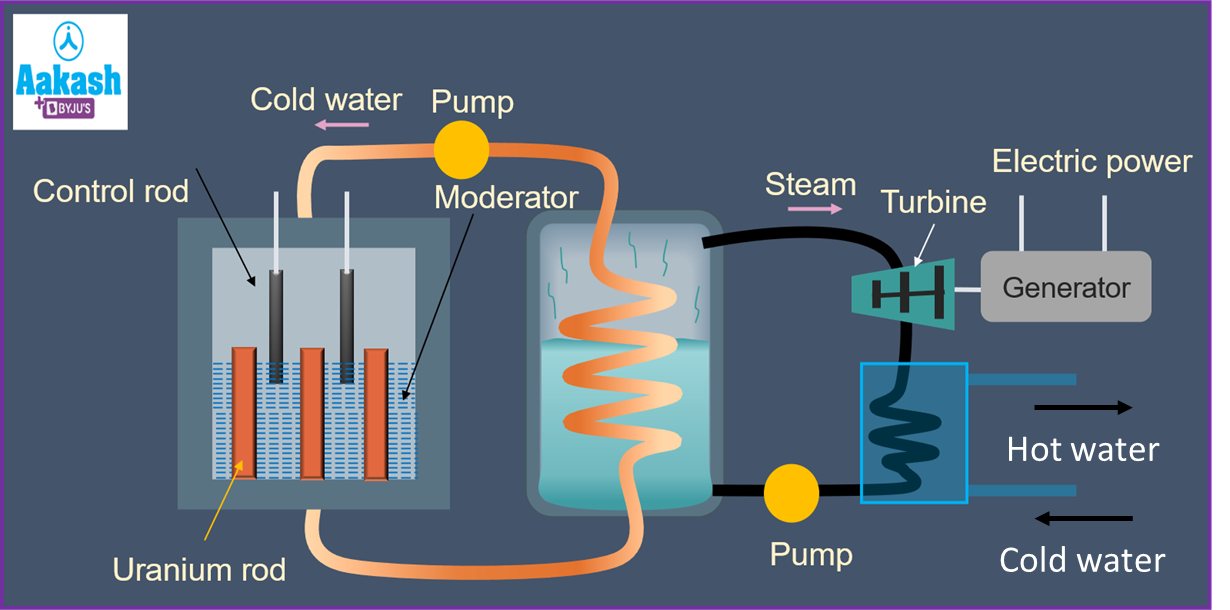1) Fuel-This is the fissionable material used in the reactor. Uranium U235, Thorium Th232, Plutonium Pu239 are commonly used as fuel.

2) Moderator: It is used to slow down the fast-moving neutrons. Graphite and heavy water D2O are used as moderators.

3) Control rods: These are cadmium rods meant for absorbing the fast-moving neutrons.

4) Coolant: Liquid is used to take away heat from the nuclear reactor core. Pressurized water is used as a coolant.

5) Shielding: A concrete wall which protects the entire reactor setup. Also prevents leakage of harmful radiation.

## Practice problems

Q1. The mass defect in a nuclear fission reaction is found to be 0.3 g. Calculate the amount of energy liberated in kilowatt-hours is (velocity of light.

(a) (b) (c) (d)

Given, mass defect,

Energy released,

Now

Hence, the energy liberated=

Q2.Which of the following is a fission reaction?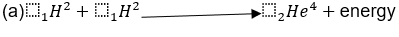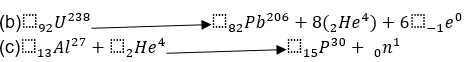(d) None of the above

Uranium 238 being unstable undergoes fission reaction.

Q3.In a nuclear reactor, U235 undergoes fission reaction liberating 200 Mega Electron Volt of energy. The efficiency of the reactor is 10 % and it generates 1000 MW power. If the reactor should function for 10 years, find the total mass of the uranium required.

Total energy to be produced in 10 years

Input energy= .

Energy produced per fission of

Number of fissions required, =Total energy liberatedEnergy per fission

Mass of uranium required

Q4.Complete the following reaction,

${}_{94}{Pu}^{239}+X$ ${\to }_{54}{Xe}^{137}{+}_{40}{Zr}^{100}+{3}_{0}{n}^{1}$

(a) (b) (c) (d)None of these

The atomic mass of the products =137+100+3=240.

The atomic mass of the reactants =239.

Atomic number of the products=94.

Hence, the required element ,i.e it is a neutron.

## FAQs

Q1.What are the there disadvantages of nuclear fission?
It is dangerous and explosive and it releases harmful products such as radiation which can harm living beings even on the genetic level.

Q2.What are the 3 uses of nuclear fission?
Answer. Nuclear fission produces electricity which can be used to run industries, schools, hospitals and homes. The leftover less radioactive materials can be used in agriculture where radiation are used to kill pests. In the medical field for imaging purposes and for destroying cancerous tissues radiation is extensively used.

Q3.Who invented nuclear fission?
Answer. Lise Meitner and Otto Frisch discovered nuclear fission.

Q4. Is nuclear fission renewable?
Answer. No, nuclear energy is not renewable. Renewable energy are those forms of energy which ultimately derive their energy from the sun or geothermal energy. Nuclear energy which we produce in nuclear power plants uses radioactive fuel which is non-renewable in nature. Though it's non-renewable in nature, nuclear energy from nuclear power plants is cleaner and more sustainable as compared to other energy sources like coal-powered or gas-powered power plants.Talk to our expert
Resend OTP Timer =
By submitting up, I agree to receive all the Whatsapp communication on my registered number and Aakash terms and conditions and privacy policy